# Video classification with a 3D convolutional neural network

This tutorial demonstrates training a 3D convolutional neural network (CNN) for video classification using the UCF101 action recognition dataset. A 3D CNN uses a three-dimensional filter to perform convolutions. The kernel is able to slide in three directions, whereas in a 2D CNN it can slide in two dimensions. The model is based on the work published in A Closer Look at Spatiotemporal Convolutions for Action Recognition by D. Tran et al. (2017). In this tutorial, you will:

• Build an input pipeline
• Build a 3D convolutional neural network model with residual connections using Keras functional API
• Train the model
• Evaluate and test the model

This video classification tutorial is the second part in a series of TensorFlow video tutorials. Here are the other three tutorials:

## Setup

Begin by installing and importing some necessary libraries, including: remotezip to inspect the contents of a ZIP file, tqdm to use a progress bar, OpenCV to process video files, einops for performing more complex tensor operations, and `tensorflow_docs` for embedding data in a Jupyter notebook.

````pip install remotezip tqdm opencv-python einops`
`# Install TensorFlow 2.10`
`pip install tensorflow==2.10.0`
```
``````import tqdm
import random
import pathlib
import itertools
import collections

import cv2
import einops
import numpy as np
import remotezip as rz
import seaborn as sns
import matplotlib.pyplot as plt

import tensorflow as tf
import keras
from keras import layers
``````
```2023-07-27 03:27:28.073979: E tensorflow/stream_executor/cuda/cuda_blas.cc:2981] Unable to register cuBLAS factory: Attempting to register factory for plugin cuBLAS when one has already been registered
2023-07-27 03:27:28.699476: W tensorflow/stream_executor/platform/default/dso_loader.cc:64] Could not load dynamic library 'libnvinfer.so.7'; dlerror: libnvinfer.so.7: cannot open shared object file: No such file or directory; LD_LIBRARY_PATH: /tmpfs/src/tf_docs_env/lib/python3.9/site-packages/cv2/../../lib64:
2023-07-27 03:27:28.699587: W tensorflow/stream_executor/platform/default/dso_loader.cc:64] Could not load dynamic library 'libnvinfer_plugin.so.7'; dlerror: libnvinfer_plugin.so.7: cannot open shared object file: No such file or directory; LD_LIBRARY_PATH: /tmpfs/src/tf_docs_env/lib/python3.9/site-packages/cv2/../../lib64:
2023-07-27 03:27:28.699596: W tensorflow/compiler/tf2tensorrt/utils/py_utils.cc:38] TF-TRT Warning: Cannot dlopen some TensorRT libraries. If you would like to use Nvidia GPU with TensorRT, please make sure the missing libraries mentioned above are installed properly.
```

## Load and preprocess video data

The hidden cell below defines helper functions to download a slice of data from the UCF-101 dataset, and load it into a `tf.data.Dataset`. You can learn more about the specific preprocessing steps in the Loading video data tutorial, which walks you through this code in more detail.

The `FrameGenerator` class at the end of the hidden block is the most important utility here. It creates an iterable object that can feed data into the TensorFlow data pipeline. Specifically, this class contains a Python generator that loads the video frames along with its encoded label. The generator (`__call__`) function yields the frame array produced by `frames_from_video_file` and a one-hot encoded vector of the label associated with the set of frames.

``````URL = 'https://storage.googleapis.com/thumos14_files/UCF101_videos.zip'
num_classes = 10,
splits = {"train": 30, "val": 10, "test": 10},
``````
```train :
100%|██████████| 300/300 [00:50<00:00,  5.98it/s]
val :
100%|██████████| 100/100 [00:13<00:00,  7.44it/s]
test :
100%|██████████| 100/100 [00:11<00:00,  8.47it/s]
```

Create the training, validation, and test sets (`train_ds`, `val_ds`, and `test_ds`).

## Create the model

The following 3D convolutional neural network model is based off the paper A Closer Look at Spatiotemporal Convolutions for Action Recognition by D. Tran et al. (2017). The paper compares several versions of 3D ResNets. Instead of operating on a single image with dimensions `(height, width)`, like standard ResNets, these operate on video volume `(time, height, width)`. The most obvious approach to this problem would be replace each 2D convolution (`layers.Conv2D`) with a 3D convolution (`layers.Conv3D`).

This tutorial uses a (2 + 1)D convolution with residual connections. The (2 + 1)D convolution allows for the decomposition of the spatial and temporal dimensions, therefore creating two separate steps. An advantage of this approach is that factorizing the convolutions into spatial and temporal dimensions saves parameters.

For each output location a 3D convolution combines all the vectors from a 3D patch of the volume to create one vector in the output volume.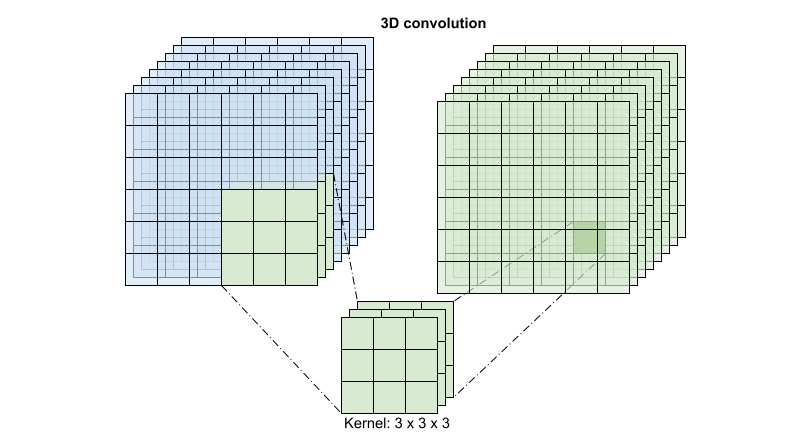This operation is takes `time * height * width * channels` inputs and produces `channels` outputs (assuming the number of input and output channels are the same. So a 3D convolution layer with a kernel size of `(3 x 3 x 3)` would need a weight-matrix with `27 * channels ** 2` entries. The reference paper found that a more effective & efficient approach was to factorize the convolution. Instead of a single 3D convolution to process the time and space dimensions, they proposed a "(2+1)D" convolution which processes the space and time dimensions separately. The figure below shows the factored spatial and temporal convolutions of a (2 + 1)D convolution.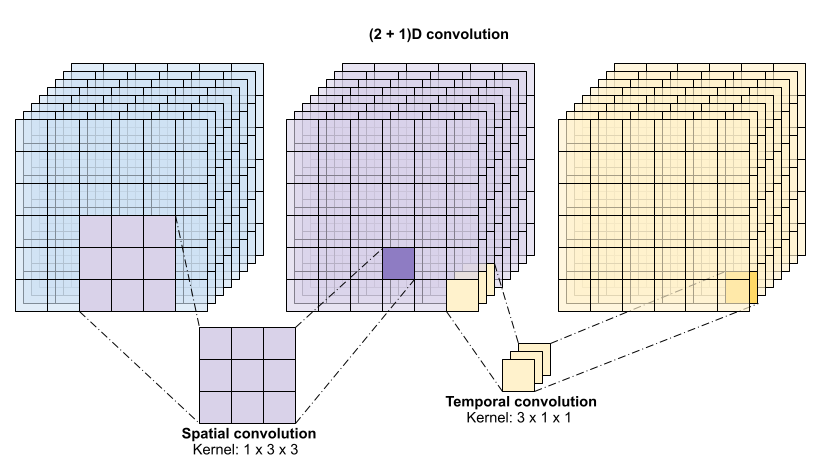The main advantage of this approach is that it reduces the number of parameters. In the (2 + 1)D convolution the spatial convolution takes in data of the shape `(1, width, height)`, while the temporal convolution takes in data of the shape `(time, 1, 1)`. For example, a (2 + 1)D convolution with kernel size `(3 x 3 x 3)` would need weight matrices of size `(9 * channels**2) + (3 * channels**2)`, less than half as many as the full 3D convolution. This tutorial implements (2 + 1)D ResNet18, where each convolution in the resnet is replaced by a (2+1)D convolution.

``````# Define the dimensions of one frame in the set of frames created
HEIGHT = 224
WIDTH = 224
``````
``````class Conv2Plus1D(keras.layers.Layer):
"""
A sequence of convolutional layers that first apply the convolution operation over the
spatial dimensions, and then the temporal dimension.
"""
super().__init__()
self.seq = keras.Sequential([
# Spatial decomposition
layers.Conv3D(filters=filters,
kernel_size=(1, kernel_size, kernel_size),
# Temporal decomposition
layers.Conv3D(filters=filters,
kernel_size=(kernel_size, 1, 1),
])

def call(self, x):
return self.seq(x)
``````

A ResNet model is made from a sequence of residual blocks. A residual block has two branches. The main branch performs the calculation, but is difficult for gradients to flow through. The residual branch bypasses the main calculation and mostly just adds the input to the output of the main branch. Gradients flow easily through this branch. Therefore, an easy path from the loss function to any of the residual block's main branch will be present. This avoids the vanishing gradient problem.

Create the main branch of the residual block with the following class. In contrast to the standard ResNet structure this uses the custom `Conv2Plus1D` layer instead of `layers.Conv2D`.

``````class ResidualMain(keras.layers.Layer):
"""
Residual block of the model with convolution, layer normalization, and the
activation function, ReLU.
"""
def __init__(self, filters, kernel_size):
super().__init__()
self.seq = keras.Sequential([
Conv2Plus1D(filters=filters,
kernel_size=kernel_size,
layers.LayerNormalization(),
layers.ReLU(),
Conv2Plus1D(filters=filters,
kernel_size=kernel_size,
layers.LayerNormalization()
])

def call(self, x):
return self.seq(x)
``````

To add the residual branch to the main branch it needs to have the same size. The `Project` layer below deals with cases where the number of channels is changed on the branch. In particular, a sequence of densely-connected layer followed by normalization is added.

``````class Project(keras.layers.Layer):
"""
Project certain dimensions of the tensor as the data is passed through different
sized filters and downsampled.
"""
def __init__(self, units):
super().__init__()
self.seq = keras.Sequential([
layers.Dense(units),
layers.LayerNormalization()
])

def call(self, x):
return self.seq(x)
``````

Use `add_residual_block` to introduce a skip connection between the layers of the model.

``````def add_residual_block(input, filters, kernel_size):
"""
Add residual blocks to the model. If the last dimensions of the input data
and filter size does not match, project it such that last dimension matches.
"""
out = ResidualMain(filters,
kernel_size)(input)

res = input
# Using the Keras functional APIs, project the last dimension of the tensor to
# match the new filter size
if out.shape[-1] != input.shape[-1]:
res = Project(out.shape[-1])(res)

``````

Resizing the video is necessary to perform downsampling of the data. In particular, downsampling the video frames allow for the model to examine specific parts of frames to detect patterns that may be specific to a certain action. Through downsampling, non-essential information can be discarded. Moreoever, resizing the video will allow for dimensionality reduction and therefore faster processing through the model.

``````class ResizeVideo(keras.layers.Layer):
def __init__(self, height, width):
super().__init__()
self.height = height
self.width = width
self.resizing_layer = layers.Resizing(self.height, self.width)

def call(self, video):
"""
Use the einops library to resize the tensor.

Args:
video: Tensor representation of the video, in the form of a set of frames.

Return:
A downsampled size of the video according to the new height and width it should be resized to.
"""
# b stands for batch size, t stands for time, h stands for height,
# w stands for width, and c stands for the number of channels.
old_shape = einops.parse_shape(video, 'b t h w c')
images = einops.rearrange(video, 'b t h w c -> (b t) h w c')
images = self.resizing_layer(images)
videos = einops.rearrange(
images, '(b t) h w c -> b t h w c',
t = old_shape['t'])
return videos
``````

Use the Keras functional API to build the residual network.

``````input_shape = (None, 10, HEIGHT, WIDTH, 3)
input = layers.Input(shape=(input_shape[1:]))
x = input

x = Conv2Plus1D(filters=16, kernel_size=(3, 7, 7), padding='same')(x)
x = layers.BatchNormalization()(x)
x = layers.ReLU()(x)
x = ResizeVideo(HEIGHT // 2, WIDTH // 2)(x)

# Block 1
x = add_residual_block(x, 16, (3, 3, 3))
x = ResizeVideo(HEIGHT // 4, WIDTH // 4)(x)

# Block 2
x = add_residual_block(x, 32, (3, 3, 3))
x = ResizeVideo(HEIGHT // 8, WIDTH // 8)(x)

# Block 3
x = add_residual_block(x, 64, (3, 3, 3))
x = ResizeVideo(HEIGHT // 16, WIDTH // 16)(x)

# Block 4
x = add_residual_block(x, 128, (3, 3, 3))

x = layers.GlobalAveragePooling3D()(x)
x = layers.Flatten()(x)
x = layers.Dense(10)(x)

model = keras.Model(input, x)
``````
``````frames, label = next(iter(train_ds))
model.build(frames)
``````
``````# Visualize the model
keras.utils.plot_model(model, expand_nested=True, dpi=60, show_shapes=True)
``````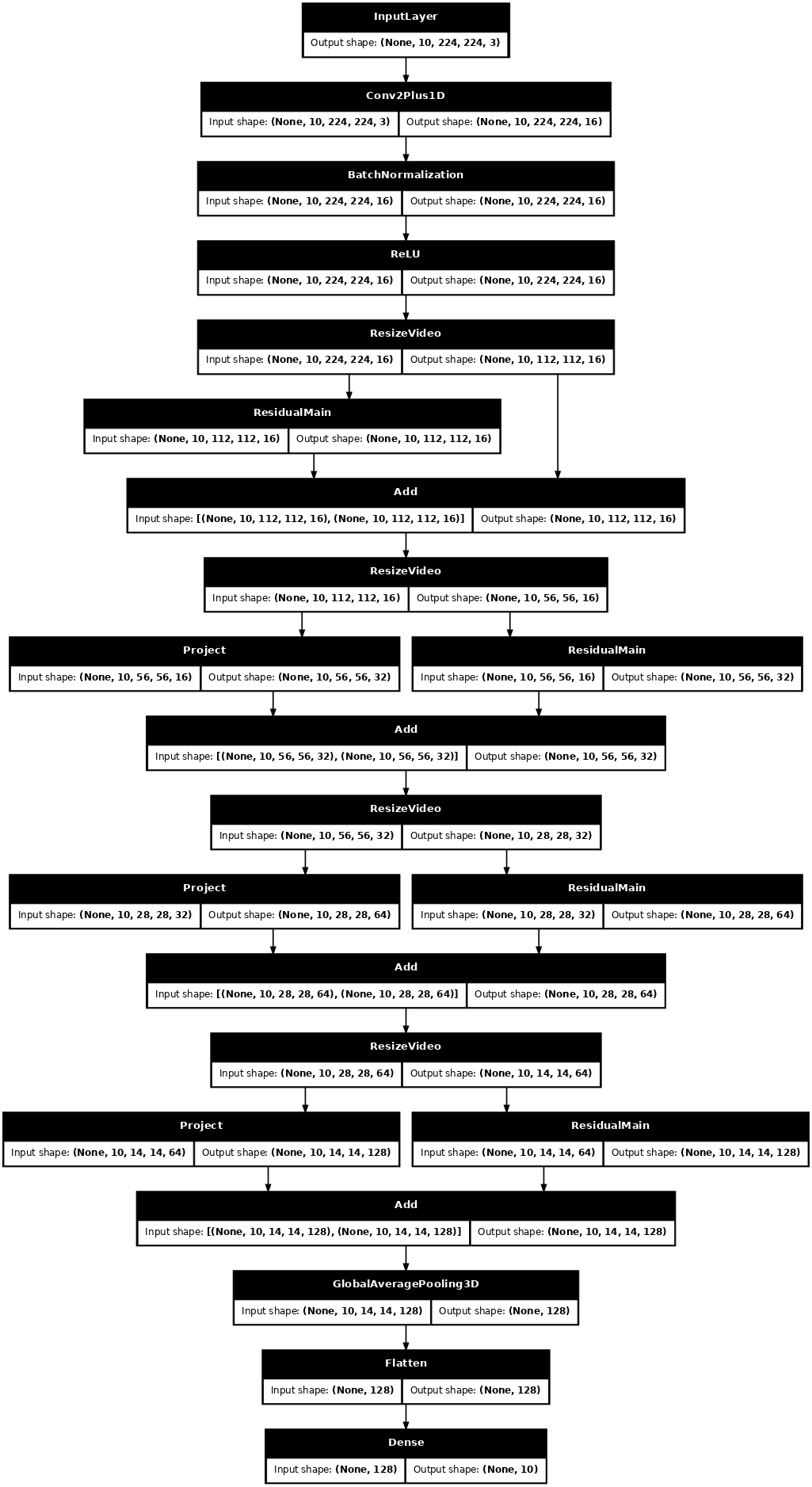## Train the model

For this tutorial, choose the `tf.keras.optimizers.Adam` optimizer and the `tf.keras.losses.SparseCategoricalCrossentropy` loss function. Use the `metrics` argument to the view the accuracy of the model performance at every step.

``````model.compile(loss = keras.losses.SparseCategoricalCrossentropy(from_logits=True),
metrics = ['accuracy'])
``````

Train the model for 50 epoches with the Keras `Model.fit` method.

``````history = model.fit(x = train_ds,
epochs = 50,
validation_data = val_ds)
``````
```Epoch 1/50
38/38 [==============================] - 66s 2s/step - loss: 2.5287 - accuracy: 0.0967 - val_loss: 2.4516 - val_accuracy: 0.1000
Epoch 2/50
38/38 [==============================] - 58s 2s/step - loss: 2.2794 - accuracy: 0.1600 - val_loss: 2.3682 - val_accuracy: 0.1500
Epoch 3/50
38/38 [==============================] - 58s 2s/step - loss: 2.1431 - accuracy: 0.2367 - val_loss: 2.1290 - val_accuracy: 0.1600
Epoch 4/50
38/38 [==============================] - 57s 2s/step - loss: 1.9739 - accuracy: 0.2867 - val_loss: 2.1153 - val_accuracy: 0.1700
Epoch 5/50
38/38 [==============================] - 58s 2s/step - loss: 1.9335 - accuracy: 0.2633 - val_loss: 2.2467 - val_accuracy: 0.1500
Epoch 6/50
38/38 [==============================] - 58s 2s/step - loss: 1.8482 - accuracy: 0.3067 - val_loss: 1.9223 - val_accuracy: 0.2900
Epoch 7/50
38/38 [==============================] - 57s 2s/step - loss: 1.7945 - accuracy: 0.2967 - val_loss: 1.9064 - val_accuracy: 0.2400
Epoch 8/50
38/38 [==============================] - 58s 2s/step - loss: 1.7885 - accuracy: 0.3400 - val_loss: 1.8131 - val_accuracy: 0.2500
Epoch 9/50
38/38 [==============================] - 57s 2s/step - loss: 1.6899 - accuracy: 0.3667 - val_loss: 2.0749 - val_accuracy: 0.2400
Epoch 10/50
38/38 [==============================] - 58s 2s/step - loss: 1.6408 - accuracy: 0.3700 - val_loss: 1.7212 - val_accuracy: 0.3500
Epoch 11/50
38/38 [==============================] - 58s 2s/step - loss: 1.5743 - accuracy: 0.4300 - val_loss: 1.7681 - val_accuracy: 0.3000
Epoch 12/50
38/38 [==============================] - 58s 2s/step - loss: 1.5412 - accuracy: 0.4300 - val_loss: 1.6751 - val_accuracy: 0.3700
Epoch 13/50
38/38 [==============================] - 58s 2s/step - loss: 1.5631 - accuracy: 0.4133 - val_loss: 1.5874 - val_accuracy: 0.4400
Epoch 14/50
38/38 [==============================] - 58s 2s/step - loss: 1.4662 - accuracy: 0.4700 - val_loss: 1.5708 - val_accuracy: 0.4100
Epoch 15/50
38/38 [==============================] - 58s 2s/step - loss: 1.3913 - accuracy: 0.4800 - val_loss: 1.6588 - val_accuracy: 0.3600
Epoch 16/50
38/38 [==============================] - 58s 2s/step - loss: 1.4170 - accuracy: 0.4967 - val_loss: 1.5524 - val_accuracy: 0.4100
Epoch 17/50
38/38 [==============================] - 58s 2s/step - loss: 1.2989 - accuracy: 0.5433 - val_loss: 1.4133 - val_accuracy: 0.4800
Epoch 18/50
38/38 [==============================] - 58s 2s/step - loss: 1.4843 - accuracy: 0.4533 - val_loss: 1.4088 - val_accuracy: 0.5100
Epoch 19/50
38/38 [==============================] - 58s 2s/step - loss: 1.2926 - accuracy: 0.5200 - val_loss: 1.5144 - val_accuracy: 0.4000
Epoch 20/50
38/38 [==============================] - 58s 2s/step - loss: 1.1462 - accuracy: 0.5867 - val_loss: 1.5705 - val_accuracy: 0.4600
Epoch 21/50
38/38 [==============================] - 58s 2s/step - loss: 1.0995 - accuracy: 0.6167 - val_loss: 1.5393 - val_accuracy: 0.4100
Epoch 22/50
38/38 [==============================] - 58s 2s/step - loss: 1.1186 - accuracy: 0.5700 - val_loss: 1.5837 - val_accuracy: 0.4400
Epoch 23/50
38/38 [==============================] - 58s 2s/step - loss: 1.1237 - accuracy: 0.5767 - val_loss: 1.2009 - val_accuracy: 0.5900
Epoch 24/50
38/38 [==============================] - 58s 2s/step - loss: 1.0716 - accuracy: 0.5867 - val_loss: 1.3369 - val_accuracy: 0.5400
Epoch 25/50
38/38 [==============================] - 58s 2s/step - loss: 1.0409 - accuracy: 0.5867 - val_loss: 1.5052 - val_accuracy: 0.4700
Epoch 26/50
38/38 [==============================] - 57s 2s/step - loss: 1.0291 - accuracy: 0.6267 - val_loss: 1.2068 - val_accuracy: 0.5500
Epoch 27/50
38/38 [==============================] - 58s 2s/step - loss: 0.9412 - accuracy: 0.6633 - val_loss: 1.2260 - val_accuracy: 0.5600
Epoch 28/50
38/38 [==============================] - 58s 2s/step - loss: 0.9516 - accuracy: 0.6833 - val_loss: 1.1595 - val_accuracy: 0.6100
Epoch 29/50
38/38 [==============================] - 57s 2s/step - loss: 0.8868 - accuracy: 0.6833 - val_loss: 1.1260 - val_accuracy: 0.6000
Epoch 30/50
38/38 [==============================] - 57s 2s/step - loss: 0.8568 - accuracy: 0.7000 - val_loss: 1.1131 - val_accuracy: 0.6300
Epoch 31/50
38/38 [==============================] - 57s 2s/step - loss: 0.8931 - accuracy: 0.6633 - val_loss: 0.9691 - val_accuracy: 0.7000
Epoch 32/50
38/38 [==============================] - 57s 2s/step - loss: 0.8779 - accuracy: 0.6533 - val_loss: 1.1402 - val_accuracy: 0.5600
Epoch 33/50
38/38 [==============================] - 58s 2s/step - loss: 0.9921 - accuracy: 0.6367 - val_loss: 1.1384 - val_accuracy: 0.5700
Epoch 34/50
38/38 [==============================] - 58s 2s/step - loss: 0.7956 - accuracy: 0.7000 - val_loss: 1.0386 - val_accuracy: 0.6700
Epoch 35/50
38/38 [==============================] - 57s 2s/step - loss: 0.8843 - accuracy: 0.6700 - val_loss: 1.1162 - val_accuracy: 0.5900
Epoch 36/50
38/38 [==============================] - 57s 2s/step - loss: 0.8088 - accuracy: 0.6867 - val_loss: 1.0426 - val_accuracy: 0.5900
Epoch 37/50
38/38 [==============================] - 58s 2s/step - loss: 0.7206 - accuracy: 0.7533 - val_loss: 1.0802 - val_accuracy: 0.5800
Epoch 38/50
38/38 [==============================] - 57s 2s/step - loss: 0.7372 - accuracy: 0.7333 - val_loss: 1.0123 - val_accuracy: 0.6700
Epoch 39/50
38/38 [==============================] - 58s 2s/step - loss: 0.6617 - accuracy: 0.7800 - val_loss: 0.9618 - val_accuracy: 0.6600
Epoch 40/50
38/38 [==============================] - 58s 2s/step - loss: 0.7664 - accuracy: 0.7167 - val_loss: 0.9366 - val_accuracy: 0.6400
Epoch 41/50
38/38 [==============================] - 58s 2s/step - loss: 0.6674 - accuracy: 0.7767 - val_loss: 0.8766 - val_accuracy: 0.6700
Epoch 42/50
38/38 [==============================] - 57s 2s/step - loss: 0.6563 - accuracy: 0.7733 - val_loss: 0.9558 - val_accuracy: 0.6600
Epoch 43/50
38/38 [==============================] - 58s 2s/step - loss: 0.6613 - accuracy: 0.7600 - val_loss: 0.9445 - val_accuracy: 0.6600
Epoch 44/50
38/38 [==============================] - 57s 2s/step - loss: 0.5847 - accuracy: 0.8100 - val_loss: 0.8455 - val_accuracy: 0.6700
Epoch 45/50
38/38 [==============================] - 58s 2s/step - loss: 0.5853 - accuracy: 0.8033 - val_loss: 1.0715 - val_accuracy: 0.6200
Epoch 46/50
38/38 [==============================] - 58s 2s/step - loss: 0.7178 - accuracy: 0.7600 - val_loss: 1.1619 - val_accuracy: 0.6400
Epoch 47/50
38/38 [==============================] - 58s 2s/step - loss: 0.6366 - accuracy: 0.7833 - val_loss: 0.8635 - val_accuracy: 0.6900
Epoch 48/50
38/38 [==============================] - 57s 2s/step - loss: 0.5157 - accuracy: 0.8133 - val_loss: 0.9152 - val_accuracy: 0.6600
Epoch 49/50
38/38 [==============================] - 58s 2s/step - loss: 0.5564 - accuracy: 0.8133 - val_loss: 1.0634 - val_accuracy: 0.6500
Epoch 50/50
38/38 [==============================] - 58s 2s/step - loss: 0.5907 - accuracy: 0.7933 - val_loss: 0.8375 - val_accuracy: 0.7100
```

### Visualize the results

Create plots of the loss and accuracy on the training and validation sets:

``````def plot_history(history):
"""
Plotting training and validation learning curves.

Args:
history: model history with all the metric measures
"""
fig, (ax1, ax2) = plt.subplots(2)

fig.set_size_inches(18.5, 10.5)

# Plot loss
ax1.set_title('Loss')
ax1.plot(history.history['loss'], label = 'train')
ax1.plot(history.history['val_loss'], label = 'test')
ax1.set_ylabel('Loss')

# Determine upper bound of y-axis
max_loss = max(history.history['loss'] + history.history['val_loss'])

ax1.set_ylim([0, np.ceil(max_loss)])
ax1.set_xlabel('Epoch')
ax1.legend(['Train', 'Validation'])

# Plot accuracy
ax2.set_title('Accuracy')
ax2.plot(history.history['accuracy'],  label = 'train')
ax2.plot(history.history['val_accuracy'], label = 'test')
ax2.set_ylabel('Accuracy')
ax2.set_ylim([0, 1])
ax2.set_xlabel('Epoch')
ax2.legend(['Train', 'Validation'])

plt.show()

plot_history(history)
``````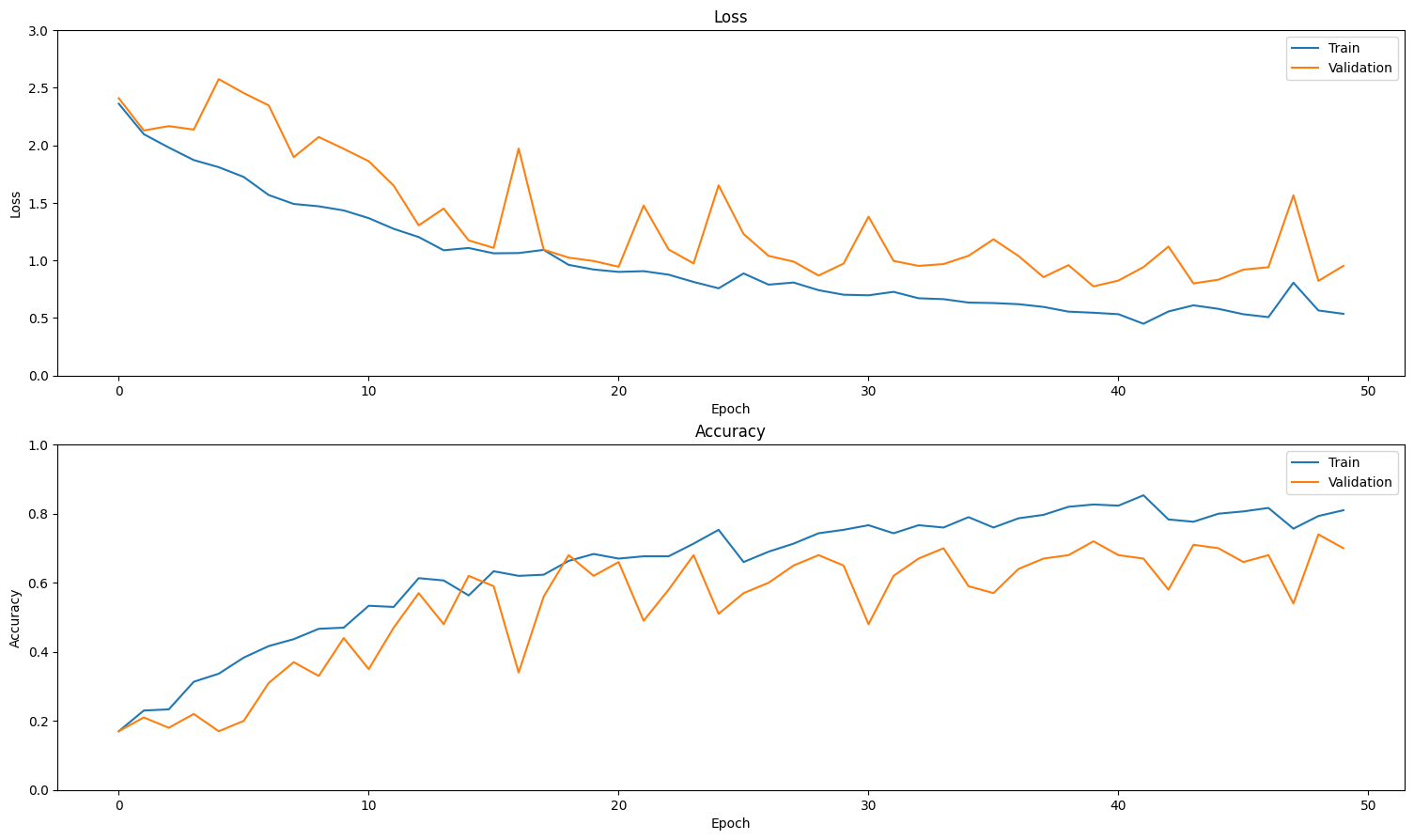## Evaluate the model

Use Keras `Model.evaluate` to get the loss and accuracy on the test dataset.

``````model.evaluate(test_ds, return_dict=True)
``````
```13/13 [==============================] - 12s 901ms/step - loss: 0.8413 - accuracy: 0.6700
{'loss': 0.8412802219390869, 'accuracy': 0.6700000166893005}
```

To visualize model performance further, use a confusion matrix. The confusion matrix allows you to assess the performance of the classification model beyond accuracy. In order to build the confusion matrix for this multi-class classification problem, get the actual values in the test set and the predicted values.

``````def get_actual_predicted_labels(dataset):
"""
Create a list of actual ground truth values and the predictions from the model.

Args:
dataset: An iterable data structure, such as a TensorFlow Dataset, with features and labels.

Return:
Ground truth and predicted values for a particular dataset.
"""
actual = [labels for _, labels in dataset.unbatch()]
predicted = model.predict(dataset)

actual = tf.stack(actual, axis=0)
predicted = tf.concat(predicted, axis=0)
predicted = tf.argmax(predicted, axis=1)

return actual, predicted
``````
``````def plot_confusion_matrix(actual, predicted, labels, ds_type):
cm = tf.math.confusion_matrix(actual, predicted)
ax = sns.heatmap(cm, annot=True, fmt='g')
sns.set(rc={'figure.figsize':(12, 12)})
sns.set(font_scale=1.4)
ax.set_title('Confusion matrix of action recognition for ' + ds_type)
ax.set_xlabel('Predicted Action')
ax.set_ylabel('Actual Action')
plt.xticks(rotation=90)
plt.yticks(rotation=0)
ax.xaxis.set_ticklabels(labels)
ax.yaxis.set_ticklabels(labels)
``````
``````fg = FrameGenerator(subset_paths['train'], n_frames, training=True)
labels = list(fg.class_ids_for_name.keys())
``````
``````actual, predicted = get_actual_predicted_labels(train_ds)
plot_confusion_matrix(actual, predicted, labels, 'training')
``````
```38/38 [==============================] - 37s 939ms/step
```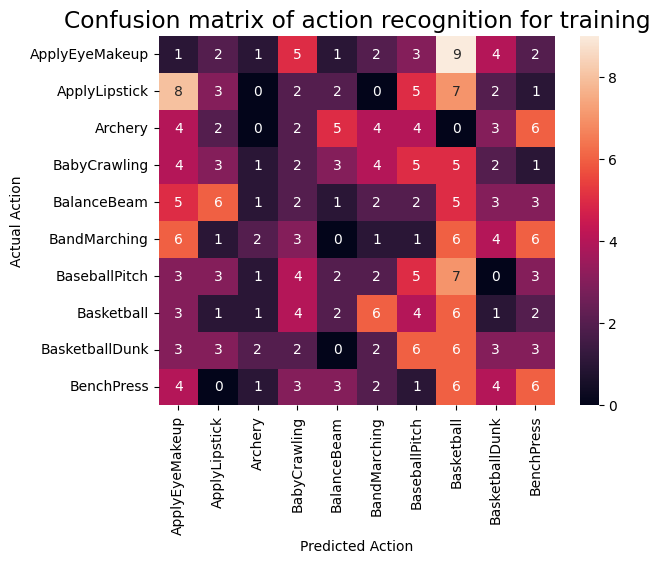``````actual, predicted = get_actual_predicted_labels(test_ds)
plot_confusion_matrix(actual, predicted, labels, 'test')
``````
```13/13 [==============================] - 12s 893ms/step
```The precision and recall values for each class can also be calculated using a confusion matrix.

``````def calculate_classification_metrics(y_actual, y_pred, labels):
"""
Calculate the precision and recall of a classification model using the ground truth and
predicted values.

Args:
y_actual: Ground truth labels.
y_pred: Predicted labels.
labels: List of classification labels.

Return:
Precision and recall measures.
"""
cm = tf.math.confusion_matrix(y_actual, y_pred)
tp = np.diag(cm) # Diagonal represents true positives
precision = dict()
recall = dict()
for i in range(len(labels)):
col = cm[:, i]
fp = np.sum(col) - tp[i] # Sum of column minus true positive is false negative

row = cm[i, :]
fn = np.sum(row) - tp[i] # Sum of row minus true positive, is false negative

precision[labels[i]] = tp[i] / (tp[i] + fp) # Precision

recall[labels[i]] = tp[i] / (tp[i] + fn) # Recall

return precision, recall
``````
``````precision, recall = calculate_classification_metrics(actual, predicted, labels) # Test dataset
``````
```/tmpfs/tmp/ipykernel_73117/791028922.py:25: RuntimeWarning: invalid value encountered in scalar divide
precision[labels[i]] = tp[i] / (tp[i] + fp) # Precision
```
``````precision
``````
```{'ApplyEyeMakeup': nan,
'ApplyLipstick': 0.3888888888888889,
'Archery': 0.45454545454545453,
'BabyCrawling': 0.75,
'BalanceBeam': 0.6666666666666666,
'BandMarching': 0.8,
'BaseballPitch': 0.9,
'BenchPress': 0.6923076923076923}
```
``````recall
``````
```{'ApplyEyeMakeup': 0.0,
'ApplyLipstick': 0.7,
'Archery': 0.5,
'BabyCrawling': 0.6,
'BalanceBeam': 1.0,
'BandMarching': 0.8,
'BaseballPitch': 0.9,